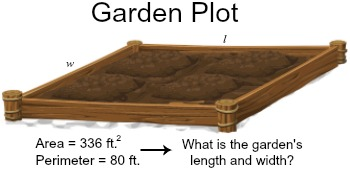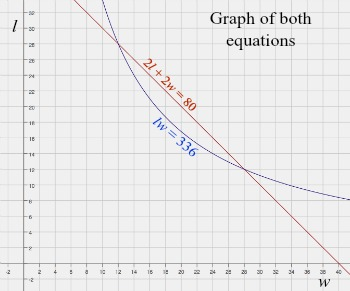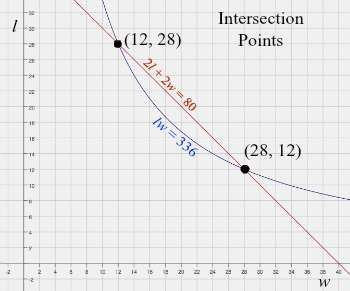# Solving Systems of Nonlinear Equations in Two Variables

Instructor: Laura Pennington

Laura has taught collegiate mathematics and holds a master's degree in pure mathematics.

In this lesson, we will take a look at how to solve non-linear systems of equations in two variables by exploring two solving techniques for these types of systems - graphing and substitution.

## Non-Linear System of Equations in Two Variables

Suppose you decide to take up gardening, but to do so, you need a garden! You decide to make one in your backyard. You want it to be rectangular, and you want it to have an area of 336 square feet.

You head to the store to buy some fencing, and a store worker says that they only have 80 feet of the fencing you want. You buy the 80 feet of fencing and head home to get started on this garden.

You have some criteria for your garden. You know that you want the area to be 336 square feet, and because you only have 80 feet worth of fencing, the distance around the rectangular garden, or the perimeter, must be 80 feet.You need to know what the length and width of the garden need to be to fit your criteria. Well, I've got good news! We're going to see how to do just this!

You know the area and the perimeter, so if we let the length be l, and the width be w, the following equations must be true.

2l + 2w = 80

lw = 336

In mathematics, a system of equations is a set of two or more equations with the same variables. When one or more of the equations in a system are non-linear, then it is called a non-linear system of equations. Notice in our system, there is a non-linear equation lw = 336, and there are two unknowns, so our system is called a non-linear system of equations in two variables.

Okay, now that we have the vocabulary out of the way, let's take a look at how to solve these types of systems so you can get started on your garden!

## Solving By Graphing

One way to solve a non-linear system of equations in two variables is by graphing. One word of caution, however! When solving by graphing, it's best to use a graphing calculator. Doing it by hand leaves a lot of room for error since solving in this way involves locating an exact point.

The steps we use to solve by graphing are as follows.

1. Graph both equations on the same graph.
2. Find the intersection point of the two graphs.
3. The intersection points are your solutions.

Okay! Three easy steps - we can handle this! Let's solve our system by graphing! The first step is to graph both equations on the same graph.Next, we locate the intersection points of the two graphs.We see the two intersection points are (12, 28) and (28, 12). The third step is the easiest! It just states that the intersection points are our solutions. In our case, we have that the length and width of the garden must be 12 feet and 28 feet to meet your criteria. Don't let the fact that there are two solutions trip you up. In this case, it just tells us that the garden will meet your criteria if it has length 12 feet and width 28 feet or length 28 feet and width 12 feet.

Awesome! You know what you need your length and width to be, but then you get to thinking, what if you didn't have a graphing calculator handy? Could you solve this system by hand another way? The answer is yes!

## Solving Using Substitution

Another way to solve non-linear systems of equations in two variables is to do so algebraically using substitution. To use substitution to solve a system in x and y, we follow these steps.

1. Solve for y in terms of x in one of the equations.
2. Plug the expression you found for y into the other equation, and solve for x.
3. Plug the values you found for x into either of the original equations to find a corresponding value of y for each value of x. These are your solutions.

To unlock this lesson you must be a Study.com Member.

### Register to view this lesson

Are you a student or a teacher?

### Unlock Your Education

#### See for yourself why 30 million people use Study.com

##### Become a Study.com member and start learning now.
Back
What teachers are saying about Study.com

### Earning College Credit

Did you know… We have over 160 college courses that prepare you to earn credit by exam that is accepted by over 1,500 colleges and universities. You can test out of the first two years of college and save thousands off your degree. Anyone can earn credit-by-exam regardless of age or education level.